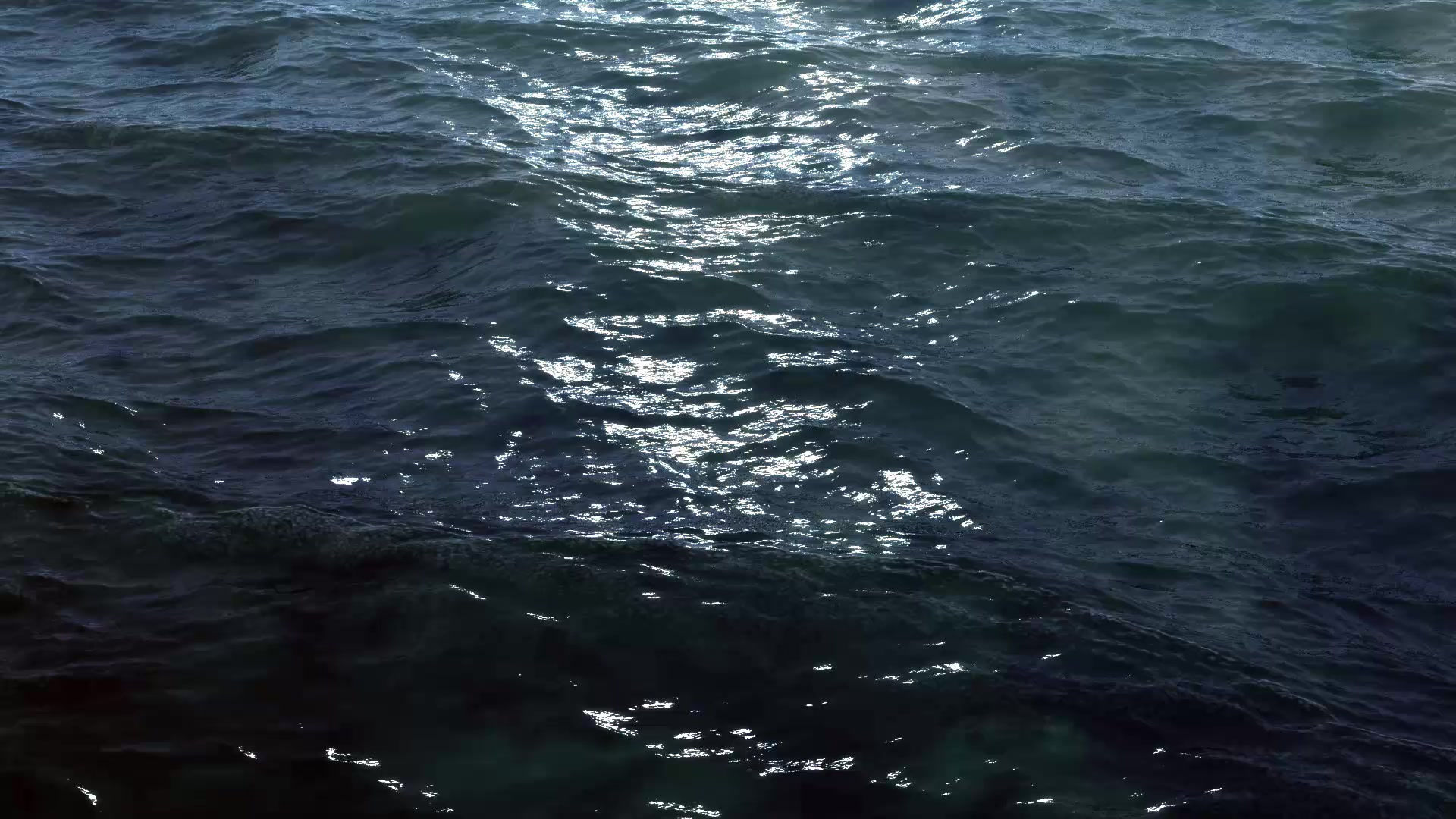top of page# Polarization vs Color for FGK dwarfsThe behaviour of degree of linear polarization P and (B − V) colour in B, V, R, and I bands is displayed in Fig. 5. The degree of polarization is found to be increasing towards later spectral type from (B − V) = 0.400 to 1.504. The correlation coefficients between BLP and (B − V) colour are found to be 0.55, 0.41, 0.37, and 0.15 in B, V, R, and

I bands, respectively. The probability of correlation is found to be more than 99 per cent in B, V, and R bands. However, the correlation in I band is found to be only 70 per cent. The slopes between P and (B − V) are found to be 0.26 ± 0.03, 0.09 ± 0.02, 0.05 ± 0.01 and 0.014 ± 0.009 in the B, V, R and I bands, respectively.

[Image Credit: Patel, Pandey, & Karmakar et al. (2016)]

bottom of page